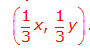# Exploring Dilations

A dilation is a type of transformation. Other transformations include translations, reflections, and rotations.

The result of a transformation is called the image. The original figure is called the pre-image.

Use the link below to explore dilations:

• Click on the ACTIVITIES button at the top of the screen.
• Follow the directions for “Playing with Dilations” that appear on the right side of the screen.
• Click on either the left or right arrow near the top right of the screen. Follow the directions for “Hitting a Target” that appear on the right side of the screen.
Playing with Dilations

After you have explored some dilations, answer the following questions in your math journal. Use the words pre-image and image in your responses.

1. When you dragged the blue slider, how did the image compare to the pre-image? What stayed the same? What changed?
2. When you rotated the pre-image, how did the image compare to the pre-image? What stayed the same? What changed?
3. When you dragged the black point on the white line (the center of dilation), how did the image compare to the pre-image? What stayed the same? What changed?
4. Use the word bank to complete the sentences below:
1. A dilation is a transformation that changes the __________ and ____________ of a figure. A dilation does not change the figure’s _____________.
2. The result of a dilation is called the ________. The original figure is called the __________.
3. If the image is smaller than the pre-image, the dilation is a __________.
4. If the image is larger than the pre-image, the dilation is a __________.

WORD BANK:

pre-image, size, enlargement, position, image, orientation, reduction

# Exploring Dilations

The result of a transformation is called the image. The original figure is called the pre-image.

Watch this video to observe the dilation of a triangle. As you watch, notice the following:

• The center of dilation is the origin.
• The term “k-factor” is used in the video. This is also known as a “scale factor.”

Respond to or complete the following in your math journal:

1. How did the x coordinates of the image compare to the corresponding x coordinates of the pre-image?
2. How did the y coordinates of the image compare to the corresponding y coordinates of the pre-image?
3. The dilation in the video was an enlargement using a scale factor of 2 and could be represented with the rule (2x, 2y). Describe the dilation represented by the rule.
4. Use the words enlargement and reduction to fill in the blanks:
If the scale factor is between 0 and 1, the dilation will be __________________.
If the scale factor is greater than 1, the dilation will be ______________________.